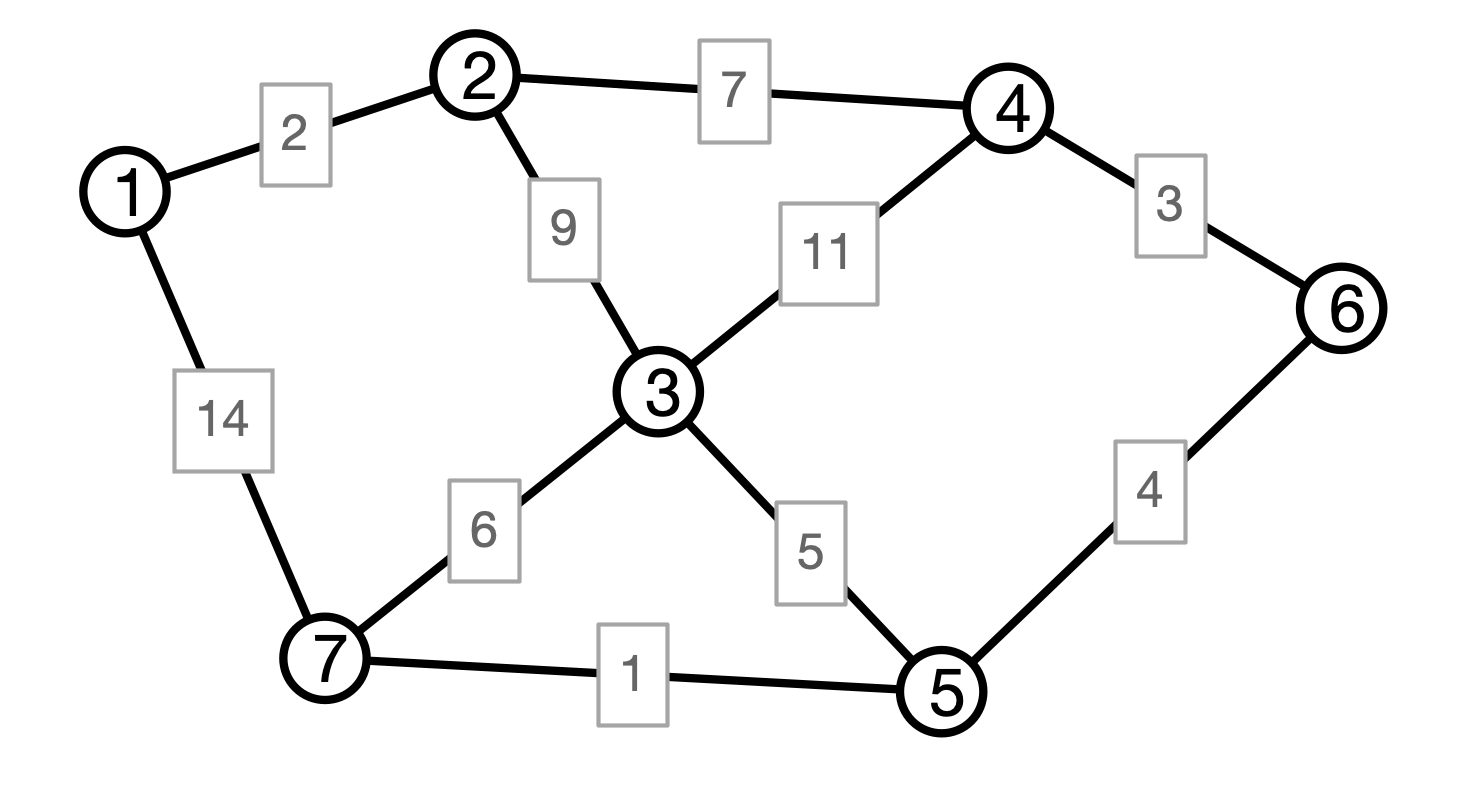# Mini Lecture: Running Time of Merging

### COSC 311 Algorithms, Fall 2022

$\def\compare{ {\mathrm{compare}} } \def\swap{ {\mathrm{swap}} } \def\sort{ {\mathrm{sort}} } \def\insert{ {\mathrm{insert}} } \def\true{ {\mathrm{true}} } \def\false{ {\mathrm{false}} } \def\BubbleSort{ {\mathrm{BubbleSort}} } \def\SelectionSort{ {\mathrm{SelectionSort}} } \def\Merge{ {\mathrm{Merge}} } \def\MergeSort{ {\mathrm{MergeSort}} } \def\QuickSort{ {\mathrm{QuickSort}} } \def\Split{ {\mathrm{Split}} } \def\Multiply{ {\mathrm{Multiply}} } \def\Add{ {\mathrm{Add}} } \def\cur{ {\mathrm{cur}} } \def\gets{ {\leftarrow} }$

## Last Time

Kruskal’s Algorithm for MSTs:

• iterate over all edges in ascending order of weight
• if an edge connects two previously un-connected components, add it to MST

## Kruskal’s Algorithm

  Kruskal(V, E, w):
C <- collection of components
initially, each vertex is own component
F <- empty collection
# iterate in order of increasing weight
for each edge e = (u, v) in E
if u and v are in different components then
merge components containing u and v
endif
endfor
return F


## Maintaining Components

Associate a leader with each component

• leader is a vertex in the component
• leader[i] = v means that v is leader of i’s component
• for each leader v, maintain a (linked) list of elements in v’s component
• list also stores size of the component

## Illustration## Merging Components

To merge components with leaders $u$ and $v$

1. Choose larger component’s leader to be new leader ($u$)
2. Iterate over each vertex $x$ in $v$’s list and
• add $x$ to $u$’s list
• update leader[x] <- u

Running time: $O(\text{size of smaller component})$

• time per element is $O(1)$

## Simplistic Analysis

  Kruskal(V, E, w):
C <- collection of components
initially, each vertex is own component
F <- empty collection
# iterate in order of increasing weight
for each edge e = (u, v) in E
if u and v are in different components then
merge components containing u and v
endif
endfor
return F


## Fewer Merges

  Kruskal(V, E, w):
C <- collection of components
initially, each vertex is own component
F <- empty collection
# iterate in order of increasing weight
for each edge e = (u, v) in E
if u and v are in different components then
merge components containing u and v
endif
endfor
return F


## Amortized Cost of Merges

Consider the number of times each element’s leader is updated

Claim. If $x$ is relabeled $k$ times, then $x$’s component has size at least $2^k$.

Consequence 1. If $x$’s component has size $\ell$, then $x$ was relabeled at most $\log \ell$ times.

Consequence 2. Running time of all merge operations in Kruskal is $O(n \log n)$

## Conclusion

Theorem. Kruskal’s algorithm can be implemented to run in time $O(m \log n)$ in graphs with $n$ vertices and $m$ edges.

• running time dominated by getting edges in ascending weight order

Remark. More efficient data structures for merging sets exist

• “Union-find” ADT, “disjoint-set forest” data structure
• time to perform merges is $O(n \alpha(n))$
• $\alpha(n)$ is “inverse Ackerman function”
• $\alpha(n)$ grows so slowly, it is practically constant